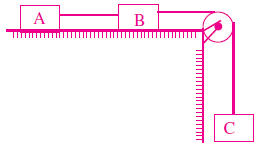Each of three blocks shown in figure has a mass 3kg. The wire connecting, blocks A and B has area of cross-section 0.005 cm2 and Young’s modulus of elasticity Y = 2 × 1011 N/m2. Neglect friction. Find the elastic potential energy stored per unit volume in wire connecting blocks A and B in steady state (in J/m2; take g = 10m/s2)

# Each of three blocks shown in figure has a mass 3kg. The wire connecting, blocks A and B has area of cross-section 0.005 cm2 and Young's modulus of elasticity Y = 2 × 1011 N/m2. Neglect friction. Find the elastic potential energy stored per unit volume in wire connecting blocks A and B in steady state (in J/m2; take g = 10m/s2)1. A

500

2. B

1000

3. C

2000

4. D

3000

Fill Out the Form for Expert Academic Guidance!l

+91

Live ClassesBooksTest SeriesSelf Learning

Verify OTP Code (required)

### Solution:

3g – T1 = 3a
T1 –T2 = 3a
T2 = 3a
=  3g = 9a
a = g/3 $⇒$T2 = 3g/3 = g = 10N

## Related content

 Difference Between Mass and Weight Differences & Comparisons Articles in Physics Important Topic of Physics: Reynolds Number Distance Speed Time Formula Refractive Index Formula Mass Formula Electric Current Formula Electric Power Formula Resistivity Formula Weight Formula+91

Live ClassesBooksTest SeriesSelf Learning

Verify OTP Code (required)